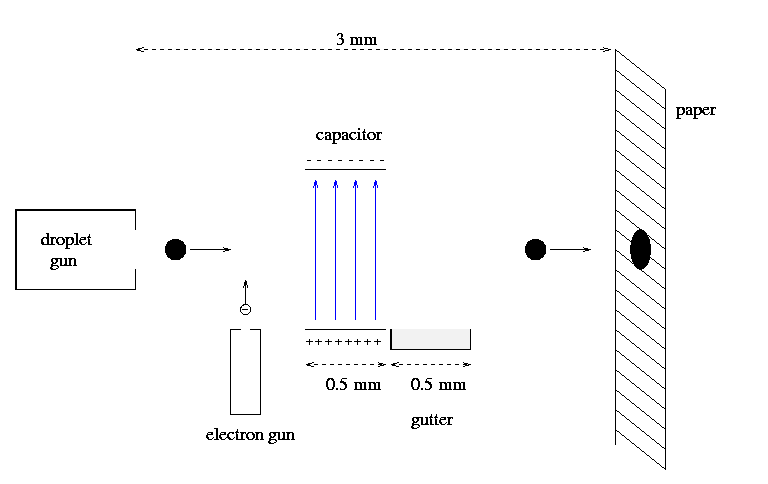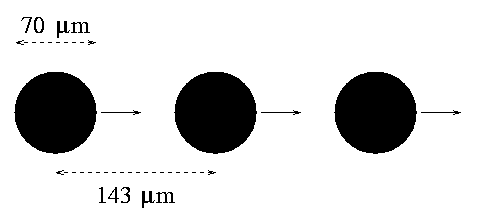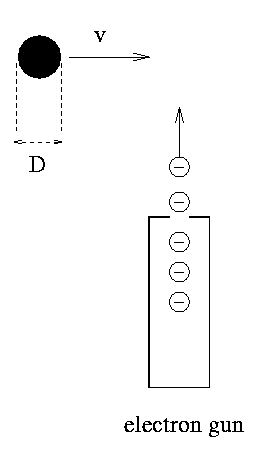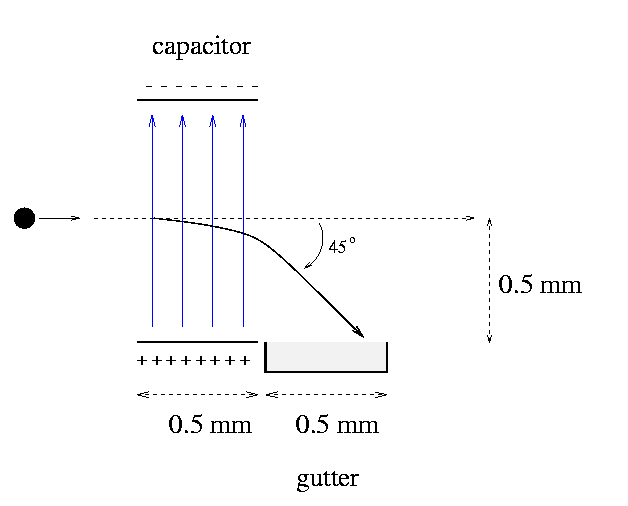Copyright © Michael Richmond. This work is licensed under a Creative Commons License.

## Physics of an Ink-Jet Printer

#### Michael Richmond March 1, 1999

Let's examine the physics involved in the mechanism of an ink-jet printer's head. The basic idea is that small droplets of ink are shot at a sheet of paper to create characters and images.The printer's head includes several different mechanisms that illustrate physical principles. We can focus in on four areas:

We'll use the following assumptions, which are reasonable, if not perhaps appropriate for any particular printer:

1. The printer produces output with 300 dots per inch; this implies that each dot on the paper is 1/300 of an inch in diameter, or 84 micrometers.
2. Droplets fly out of the ink gun and strike the paper at a speed of 20 meters per second.

#### The Ink Droplets

Let us assume that each droplet of ink is a sphere of diameter D as it flies towards the paper, then creates a spherical dot about 20 percent larger than D when it strikes the paper. How large must the drop be to yield a resolution of 300 dots per inch?

```       each dot = 1/300 inch = 84 micrometers (microns)

-> 84 microns = 1.20 D

->          D = 70 microns
radius R = 35 microns
```

We can derive a number of properties from the size of the drop:

```
4      3              -13   3
volume      V = --- pi R   =  1.13 x 10     m
3
```
Assume that the density of ink is about the same as that of water, 1000 kg/m^3.
```       mass        M = (density)      *    (volume)

= (1000 kg/m^3)  *  (1.13 x 10^(-13) m^3)

= 1.13 x 10^(-10) kg
```
How many atoms are in the drop? For simplicity, we make the very rough approximation that the drop is pure carbon (although it's really a suspension of carbon atoms in a liquid of some sort).
```      mass of 1 carbon atom   m  =   12 atomic mass units

=   12 (1.66 x 10^(-27) kg)

mass of drop
number of atoms in drop Na =  ----------------------
mass of one atom

1.13 x 10^(-10) kg
=  ----------------------
1.99 x 10^(-26) kg

=   5.7 x 10^15   atoms
```

The printer shoots droplets horizontally towards the paper. When aiming for a particular spot on the paper, is it necessary to take into account the deflection of the drops due to gravity during the flight? Well, how far does the droplet fall downwards during its flight?

```       distance to paper      d  =   3 mm

speed of droplet       v  =  20 m/s

d
time to reach paper    t  = ----- =  1.5 x 10^(-4) s
v

drop falls down        h  = 0.5 * g * t^2

2
= 0.5 * (9.8 m/s^2) * (1.5 x 10^(-4) s)

= 1.1 x 10^(-7) m

= 0.11 microns
```
Now, since the droplet itself has a radius of R = 35 microns, this deflection is only a tiny fraction of the drop's size. We can ignore the effect of gravity on the drop without incurring any perceptible change in the placement of dots on the paper.

#### The Ink Gun

Let's examine two properties of the gun: how much energy it must spend in shooting drops, and how many drops per second it must expel.

The ink gun must shoot droplets horizontally towards the paper at a speed of v = 20 m/s. How much kinetic energy does each drop contain?

```                                            2
KE of one drop      =  0.5 * M * v

2
=  0.5 * (1.13 x 10^(-10) kg) * (20 m/s)

=  2.3 x 10^(-8)  Joules
```

How many drops must the ink jet produce each second? It depends on how fast the printer must create finished pages. Suppose that the goal is one page in 60 seconds. The most difficult page to print is one which is covered completely in black ink. Such a page would require

```        number of droplets    = (number of dots to cover page horizontally) *
(number of dots to cover page vertically)

8.5 inches        11 inches
=  -------------  *  ------------
300 dpi             300 dpi

=  8.4 x 10^6 droplets
```
In order to print the page in 60 seconds, the ink jet must spit out
```                                  8.4 x 10^6 droplets
number of drops per second  =  ---------------------
60 seconds

=   140,000 drops per second
```

The total kinetic energy of all these droplets can be expressed as a power consumed by the ink jet:

```                      power   =  (KE per drop) * (number of drops per second)

=  (2.3 x 10^(-8) J) * (140,000 drops per second)

=  0.03 watts
```
Evidently, a single ink gun requires very little power.

In order to print a page more rapidly, one might mount a number of ink guns on a single head. Some ink jet printers have 30 guns per head; they might be able to print a single page in two seconds.

How much space lies between each of the droplets as they fly through the air towards the paper? Each droplet takes t = 0.00015 seconds to cover the gap between head and paper. During that time, there must be

```      number of drops in air  =  t * (number of drops per second)

=  (0.00015 sec) * (140,000 drops per sec)

=  21 drops
```
simultaneously between the head and paper. If they are equally spaced, then the distance between each drop is
```                                    3 mm
distance between drops  =  ------------
21 drops

=  143 microns

=  approx. 2 times drop diameter
```How much ink does the printer use? The simplest, but most wasteful, method of operation is to produce ink droplets continuously during the printing process. Suppose that a printer does so -- how much ink does it consume per second?

```         mass of ink          =  (mass of one drop) * (number of drops)

=  (1.13 x 10^(-10) kg) * (140,000 drops per sec)

=  1.6 x 10^(-5) kg per sec

=  0.016 grams per sec
```

How long does a typical ink-jet cartridge last? Let's do a very rough, order-of-magnitude estimate. Suppose a printer is active for two minutes per hour, 8 hours a day, 5 days a week. Then each week, it runs for 80 minutes. During that time, it uses a total of

```                                 0.016 grams                  60 sec
mass of ink per week =  -----------  * 80 minutes *  -------
second                     minute

=  80 grams per week
```

Real ink jets (probably) have intelligent algorithms which turn off the ink gun when it's not needed for a while (between words, or between characters). They are more efficient than the simple-minded printer in this example.

#### The Electron Gun

In order to control the motion of ink droplets, they must be given an electric charge. The electron gun can shoot electrons at a droplet as it moves past the gun in order to give it a negative electric charge. How much current does it take?The droplet has a diameter D = 70 microns, and moves with a speed v = 20 m/s past the electron gun. Electrons fired straight out of the gun will hit the droplet during a window of time
```                                 diameter of droplet
window of time     T  =  -------------------
speed of droplet

70 x 10^(-6) m
=  --------------
20 m/s

=  3.5 x 10^(-6) s
```

The electron gun must give the droplet a charge of about q = 1.9 x 10^(-10) Coulombs in order to permit the capacitor to deflect it into the gutter. Assuming that all electrons which strike the droplet are absorbed into it, the current of electron beam must therefore be

```                                  electric charge
current of beam       =  -----------------
window of time

1.9 x 10^(-10) C
=  ------------------
3.5 x 10^(-6) s

=   5.4 x 10^(-5) amp

=   54  microamps
```

Of course, some of the droplets must not be charged. The electron gun must be able to switch on and off at precise intervals. Specifically, the gun must turn on and off in the amount of time between one drop and the next. The space between drops is about 143 microns, so the time interval between drops -- during which the electron gun must be able to switch on/off -- is

```                                 distance between drops
switching time         =  ----------------------
speed of drops

143 x 10^(-6) m
=  --------------------
20 m/s

=   7.1 microseconds
```

How many electrons does it take to give a droplet the requisite charge?

```                                    charge per drop
number of electrons   Ne =  ---------------------
charge per electron

1.9 x 10^(-10) C
=  ---------------------
1.6 x 10^(-19) C

=   1.2 x 10^9  electrons
```

Recall that a drop consists of about Na = 5.7 x 10^15 atoms. So the ratio of "extra" electrons to atoms is very small:

```                                  Ne
ratio of free electrons   =  ----
Na

1.2 x 10^9 electrons
=  ---------------------
5.7 x 10^15 atoms

=   2.1 x 10^(-7)

```
In other words, about one atom out of every 5 million has an "extra" electron when the drop is fully charged.

#### The Deflecting Capacitor

The ink gun sends a steady stream of droplets towards the paper. If they all reached the paper, they would leave a solid black area. In order to form letters, numbers, and images, the printer must somehow prevent some of the droplets from reaching the paper; only a small fraction of all the droplets strike the paper for a typical document.

The capacitor performs this task: it creates a uniform electric field between its plates. Neutral droplets pass through the field unaffected and strike the paper. Droplets with a negative charge, however, are deflected downwards (towards the positive plate) and fall into a reservoir called the gutter.

How strong must the electric field be in order to deflect charged droplets strongly enough to force them into the gutter? We know

```
droplet mass         M  =  1.13 x 10^(-10) kg

droplet charge       q  =  1.9 x 10^(-10) Coulombs

horizontal speed     v  =  20 m/s

length of capacitor  L  =  0.5 mm

height of capacitor  H  =  1.0 mm

length of gutter     x  =  0.5 mm
```

If a droplet has a velocity directed downwards at a 45 degree angle when it leaves the capacitor, then it will move downwards by 0.5 mm (the distance from the original path to the gutter) in the same time that it moves forwards by 0.5 mm (the distance from the right edge of the capacitor to the end of the gutter).How strong must the electric field be to impart this 45 degree angle?

```         horizontal speed           =  20 m/s

-> final vertical speed    =  20 m/s

original vertical speed =   0 m/s

-> change in vert. speed   =  (20 - 0) = 20 m/s
```

How long is the droplet inside the capacitor?

```                                       length of capacitor
time inside capacitor    t =  --------------------
speed of droplet

0.5 mm
=  -----------
20 m/s

=  2.5 x 10^(-5) seconds

```

So the acceleration in the vertical direction must be

```                                       (change in speed)
vertical acceleration    a =  ------------------
time

20 m/s
=  ---------------
2.5 x 10^(-5) s

=  8 x 10^5  m/s^2
```

Now we know how strong the electric force must be to provide this acceleration, and can calculate from it the stregth of the electric field within the capacitor:

```
electric force        =   (mass) * (acceleration)

q * E             =      M   *   a

M * a
->                  E  =  -------
q

(1.13 x 10^(-10) kg) * (8 x 10^5 m/s^2)
E  =  ---------------------------------------
1.9 x 10^(-10) Coulomb

E  =  4.8 x 10^5  N/C

E  =  4.8 x 10^5  Volts/meter
```

Since the plates of the capacitor are a distance 1 mm apart, the voltage between them must be

```       voltage between plates    V  =  (4.8 x 10^5 V/m) * (0.001 m)

=   480 volts
```

This is strong enough to give a person a nasty shock.Copyright © Michael Richmond. This work is licensed under a Creative Commons License.